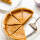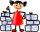# Fraction calculator

The calculator performs basic and advanced operations with fractions, expressions with fractions combined with integers, decimals, and mixed numbers. It also shows detailed step-by-step information about the fraction calculation procedure. Solve problems with two, three, or more fractions and numbers in one expression.

## Result:

### 57/8 * 18/4 = 423/16 = 26 7/16 = 26.4375

Spelled result in words is four hundred twenty-three sixteenths (or twenty-six and seven sixteenths).

### How do you solve fractions step by step?

1. Conversion a mixed number 5 7/8 to a improper fraction: 5 7/8 = 5 7/8 = 5 · 8 + 7/8 = 40 + 7/8 = 47/8

To find new numerator:
a) Multiply the whole number 5 by the denominator 8. Whole number 5 equally 5 * 8/8 = 40/8
b) Add the answer from previous step 40 to the numerator 7. New numerator is 40 + 7 = 47
c) Write a previous answer (new numerator 47) over the denominator 8.

Five and seven eighths is forty-seven eighths
2. Multiple: 47/8 * 18/4 = 47 · 18/8 · 4 = 846/32 = 423 · 2/16 · 2 = 423/16
Multiply both numerators and denominators. Result fraction keep to lowest possible denominator GCD(846, 32) = 2. In the next intermediate step, , cancel by a common factor of 2 gives 423/16.
In words - forty-seven eighths multiplied by eighteen quarters = four hundred twenty-three sixteenths.

#### Rules for expressions with fractions:

Fractions - use the slash “/” between the numerator and denominator, i.e., for five-hundredths, enter 5/100. If you are using mixed numbers, be sure to leave a single space between the whole and fraction part.
The slash separates the numerator (number above a fraction line) and denominator (number below).

Mixed numerals (mixed fractions or mixed numbers) write as non-zero integer separated by one space and fraction i.e., 1 2/3 (having the same sign). An example of a negative mixed fraction: -5 1/2.
Because slash is both signs for fraction line and division, we recommended use colon (:) as the operator of division fractions i.e., 1/2 : 3.

Decimals (decimal numbers) enter with a decimal point . and they are automatically converted to fractions - i.e. 1.45.

The colon : and slash / is the symbol of division. Can be used to divide mixed numbers 1 2/3 : 4 3/8 or can be used for write complex fractions i.e. 1/2 : 1/3.
An asterisk * or × is the symbol for multiplication.
Plus + is addition, minus sign - is subtraction and ()[] is mathematical parentheses.
The exponentiation/power symbol is ^ - for example: (7/8-4/5)^2 = (7/8-4/5)2

#### Examples:

adding fractions: 2/4 + 3/4
subtracting fractions: 2/3 - 1/2
multiplying fractions: 7/8 * 3/9
dividing Fractions: 1/2 : 3/4
exponentiation of fraction: 3/5^3
fractional exponents: 16 ^ 1/2
adding fractions and mixed numbers: 8/5 + 6 2/7
dividing integer and fraction: 5 ÷ 1/2
complex fractions: 5/8 : 2 2/3
decimal to fraction: 0.625
Fraction to Decimal: 1/4
Fraction to Percent: 1/8 %
comparing fractions: 1/4 2/3
multiplying a fraction by a whole number: 6 * 3/4
square root of a fraction: sqrt(1/16)
reducing or simplifying the fraction (simplification) - dividing the numerator and denominator of a fraction by the same non-zero number - equivalent fraction: 4/22
expression with brackets: 1/3 * (1/2 - 3 3/8)
compound fraction: 3/4 of 5/7
fractions multiple: 2/3 of 3/5
divide to find the quotient: 3/5 ÷ 2/3

The calculator follows well-known rules for order of operations. The most common mnemonics for remembering this order of operations are:
PEMDAS - Parentheses, Exponents, Multiplication, Division, Addition, Subtraction.
BEDMAS - Brackets, Exponents, Division, Multiplication, Addition, Subtraction
BODMAS - Brackets, Of or Order, Division, Multiplication, Addition, Subtraction.
GEMDAS - Grouping Symbols - brackets (){}, Exponents, Multiplication, Division, Addition, Subtraction.
Be careful, always do multiplication and division before addition and subtraction. Some operators (+ and -) and (* and /) has the same priority and then must evaluate from left to right.

## Fractions in word problems:

• DividendsThe three friends divided the win by the invested money. Karlos got three-eighths, John 320 permille, and the rest got Martin. Who got the most and which the least?
• BuingBrother got to buy 240 CZK and could buy for 1/8 what he wanted. Could he pay the rest of the purchase for 200 CZK?
• SandySandy, John and Marg baked pies for the Bake Sale. Sandy cut his pies into 6ths, John but his into 8ths and Marg cut hers into quarters. Sandy sold 11/6, John sold  1 3/8 pies and Marg sold 9/4 pies. Who sold the most pies? Who sold the fewest?
• Pumpkin pieHave some pumpkin pie. One half of the pie is cut into 4 equal slices, the other half is cut into 3 equal slices. After eating one of the larger slices (on the 3 piece side), I wonder if I will eat more if I have one more from the 3 side, or two from the
• Colored blocksTucker and his classmates placed colored blocks on a scale during a science lab. The brown block weighed 8.94 pounds, and the red block weighed 1.87 pounds. How much more did the brown block weigh than the red block?
• Engineer KažimírThe difference between politicians-demagogues and reasonable person with at least primary education beautifully illustrated by the TV show example. "Engineer" Kažimír says that during their tenure there was a large decline in the price of natural gas, pri
• MeadowOn the meadow grazing horses, cows, and sheep, together with less than 200. If cows were 45 times more, horses 60 times more, and sheep 35 times more than there are now, their numbers would equally. How many horses, cows, and sheep are on the meadow toget
• Ten fractionsWrite ten fractions between 1/3 and 2/3
• Paper collectingAt the paper collecting contest gathered Franta 2/9 ton, Karel 1/4 ton, and Patrick 19/36 tons of paper. Who has gathered the most and the least?
• Comparing and sortingArrange in descending order this fractions: 2/7, 7/10 & 1/2
• Pizza palaceJosh is at Enzo's pizza palace. He can sit at a table with 5 of his friends or at a different table with seven of his friends. The same size pizza is shared equally among the people at each table. At which table should Josh sit to get more pizza? (write a
• Stones in aquariumIn an aquarium with a length 2 m; width 1.5 m and a depth of 2.5 m is a water level up to three-quarters of the depth. Can we place stones with a volume of 2 m3 into the aquarium without water being poured out?
• TroopsThe route is long 147 km and the first-day first regiment went at an average speed of 12 km/h and journey back 21 km/h. The second day went second regiment the same route at an average speed of 22 km/h there and back. Which regiment will take route longer